# Algebra 1 : How to subtract integers

## Example Questions

1 2 3 5 Next →

### Example Question #68 : Real Numbers

Subtract the numbers: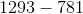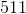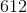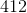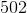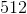Explanation:

Subtract the ones digits.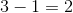Subtract the tens digits.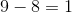Borrow a one from the thousands place in order to subtract the hundreds digits.  The thousands digit will become zero.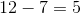Combine the numbers in order from hundreds place, tens place, and ones place.

The answer is:### Example Question #69 : Real Numbers

Subtract the numbers: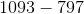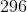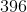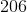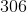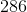Explanation:

Borrow a one from the tens place to subtract the ones digits.  The tens digit of 1093 is now eight.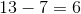We cannot borrow a one from the hundreds place to subtract eight with nine, so we will need to borrow a one from the thousands place instead.  The thousands place of 1093 is now zero, and the hundreds place becomes a nine.

Subtract the tens places.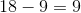Subtract the hundreds places.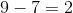Combine the hundreds, tens, and ones digits.

The answer is:1 2 3 5 Next →